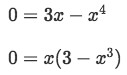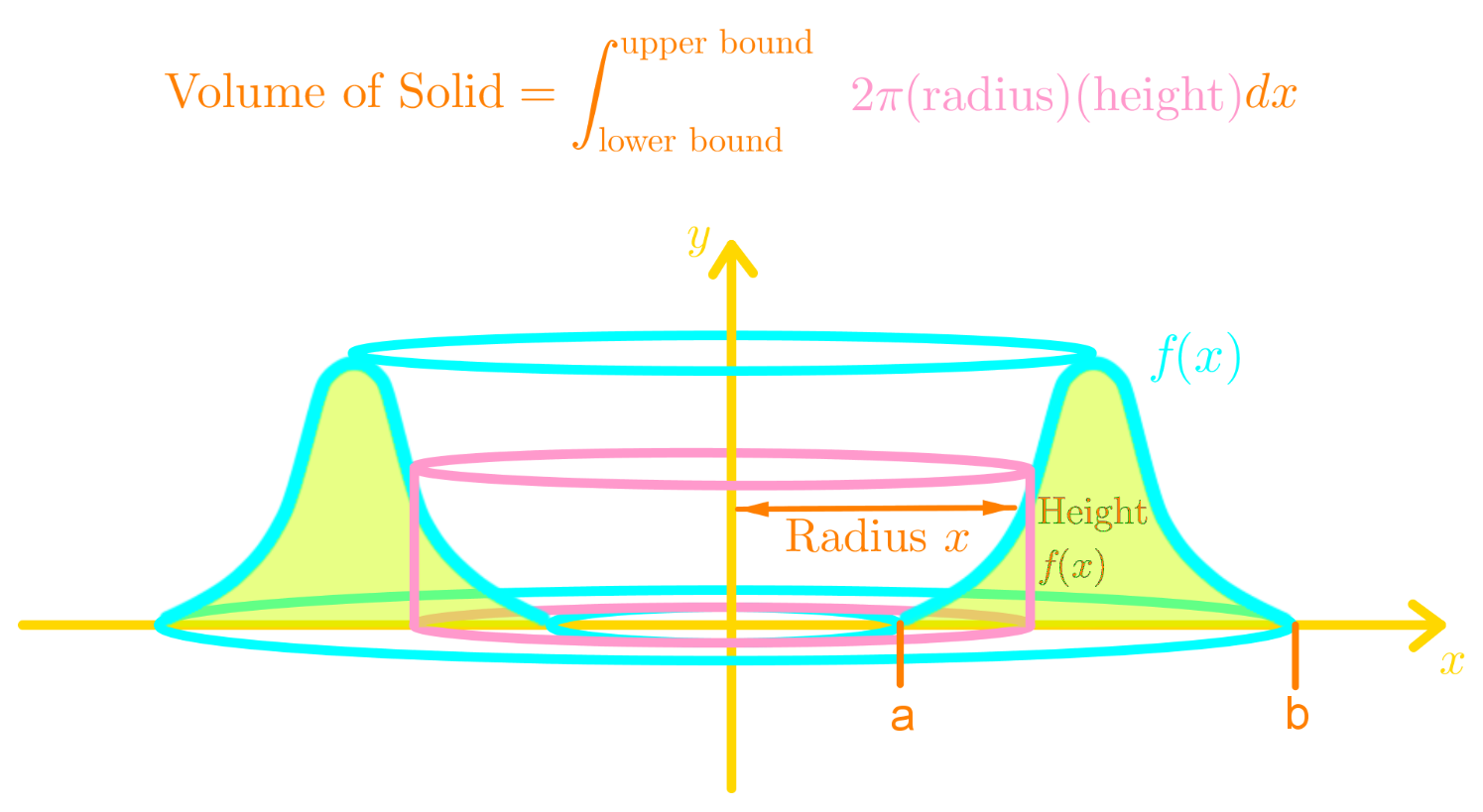# Volumes of solid of revolution - Shell method

## Shell Method

Shell method (also known as the method of cylindrical shells) is another method that is used in finding the volume a solid. To be more precise, shell method is used when the rotation of the function creates cylinder-like shells as the cross section. Once you get the area of the cylindrical shells, then integrating it will give us the volume of the solid. It is similar to the disk method and washer method because it involves solids of revolution, but the process in using shell's method is slightly different. The Shell Method formula is one of:

where r is the radius and h is the height. The first one is used for shell method around y axis, and the second one is shell method around x axis. Shell method can even be used for rotations around specific x and y values.

The interesting thing is that if we were to integrate the area of the cylindrical shell, then we actually get the volume of cylinder. Look at

Ignoring the constant of integration, we see πr2 h is the cylinder volume formula.

Now here is a question you are probably asking. Why do we introduce another method? This is because there some cases where the disc method (or washer method) actually fails, and cannot calculate the volume of a solid. Let me give you an example.

## Shell Method With Y Axis Rotation

Question 1: Find the volume of the solid obtained by rotating the region bounded by the x-axis and the following curve about the y-axis.

Since we are dealing with two functions (x-axis and the curve), we are going to use the washer method here. Recall that the washer method formula for y-axis rotation is:

Where outer is the outer radius of the circle, and inner is the inner radius of the circle.

Notice that the curve looks like this:

Rotating it around the y-axis will show us the inner radius and outer radius

However, notice that both the inner radius and outer radius occur on the same function. So if we were to use the washer-method formula, then

Obviously the volume is not 0, so the washer method fails here. Now let's actually do the question with shell method.

• Since the region is bounded by the x-axis and the curve, then we would like to find the intersection to get the bounds. Notice that the x-axis is the equation

• So setting y=0 and the curve equal gives:• We see that the solutions for x are

And

• So our lower bound will be a 0 and the upper bound will be the cube root of 3. Now drawing the curves and plotting those bounds gives us the following graph:

• Now what were going to do is rotate this around the y-axis, and show you how to imagine the cylindrical shell.

• Notice the slice of cylindrical shell in the middle. Of course that is not the only cylindrical shell you can draw, you can actually have an infinite amount of shells stacked together. The point is that if you were to stack all these cylindrical shells, then you would get the volume of the solid. Recall that shell method formula for around the y axis is

Notice how the radius of the cylinder depends on x. In addition, the height of the cylinder is between the x-axis and the curve. Hence we can say that:

• Plugging everything in our shell method formula gives us:

• Factoring 2π out of the integral and distributing x gives:

• Integrating and evaluating gives:

So this is how shell method integration looks like around the y-axis, but what around the x-axis? Let's do a question with that.

## Shell Method With X Axis Rotation

Question 2: Find the volume of the solid by rotating the positive region bounded by

• First, let's take a look at the region we are bounded by:

• Note that the formula for the shell method around x-axis is:

• Notice the dy here. This means we have to make sure that everything is in terms of y. So changing our curve in terms of y will lead us to have:

• Our upper bound and lower bound also have to be in terms of y. Looking at the region we just drew we see that the lower bound is at y=0, and the upper bound is the intersection between x=27 and x=y^3. Solving for the intersection we see that:

• So the upper bound is 3. Now what is the radius and height? We need to look at what the graph looks like when it is rotated around the x axis.

• Notice that the cylindrical shell is now sideways. The radius of the circle depends on y (r=y), and the height of the cylindrical shell is between

So the height would be the subtraction of the two. In other words,

• Now we have everything to plug into the formula.

• Factoring 2π out of the integral and distributing the y we have:

• Integrating and evaluating we have that:

which is the volume of the solid.

Note that this question can also be solved from using the disk method. Recall the disk method formula for x-axis rotations

• The bounds are different here because they are in terms of x. However from the graph we see that the lower bound is x=0 and upper bound is x=27. So plugging everything we have:

• Factoring π out of the integral and multiplying the exponents we have:

• Integrating gives us:

• Evaluating will give us:

As you can see, we get the same answer. Hence, both shell and disk method works here.

## Shell Method with X-Value Rotation

Let's take a look at a question which involves rotation around a specific x value.

Question 3: Find the volume of the solid obtained by rotating the positive region bounded by

• First of all, let's draw the curves and see where the region is.

• So that is the positive region, but what are the bounds in integrating this region? We need to find the intersections of the curves to determine that. Setting the two curves equal to each other we have:

However notice that we are only dealing with positive regions, so we are going to exclude x=-1. Hence, the two x values are x=0, and x=1.

• Note when x=0, then

And when x=1, then

• So we have the points (0,0) and (1,1). Putting these in our graph we will have the following:

• Now we are going to rotate this graph around x=2.

You can see that from the rotation, we can visualize cylindrical shells that are facing upward. We can also see that radius of this cylinder will be difference of 2 and the y=x curve. The height of cylinder will be the difference between the two curves. In other words,

• If you didn't understand why the radius and height are those equations, then you might be able to see it in the next graph.

• Notice how everything is in terms of x. Therefore our lower bounds and upper bounds should be in terms of x as well. So in this case, the lower bound is x=0 and the upper bound is x=1. Recall the shell method formula in terms of x is:

• Plugging everything in we have:

• Factoring 2π out of the integral and distributing we have:

• Integrating and evaluating gives:

which is the volume of the solid. Keep in mind that this is also solvable with washer's method.

If you want to look at more examples of finding the volume of solid using the shell method, then I suggest you look at this link.

http://tutorial.math.lamar.edu/Classes/CalcI/VolumeWithCylinder.aspx

Paul covers a lot of questions using the shell method

### Volumes of solid of revolution - Shell method

#### Lessons• Introduction

• 1.
Find the volume of the solid obtained by rotating the region bounded by the x-axis and $y=3x-x^4$ about the y-axis

• 2.
Find the volume of the solid obtained by rotating the positive region bounded by $y=x^3$, $y=x$
a)
about $x=2$
about $x=-2$
Use the Shell Method to find the volume of the solid obtained by rotating about the x-axis the region under $y=x^3$ from $x=0$ to $x=2$